signed

QiShunwang

“诚信为本、客户至上”

# 通信系统未编码、卷积码与格雷码的仿真性能比较

2021/5/14 21:40:21   来源：

## 通信系统未编码、卷积码与格雷码的仿真性能比较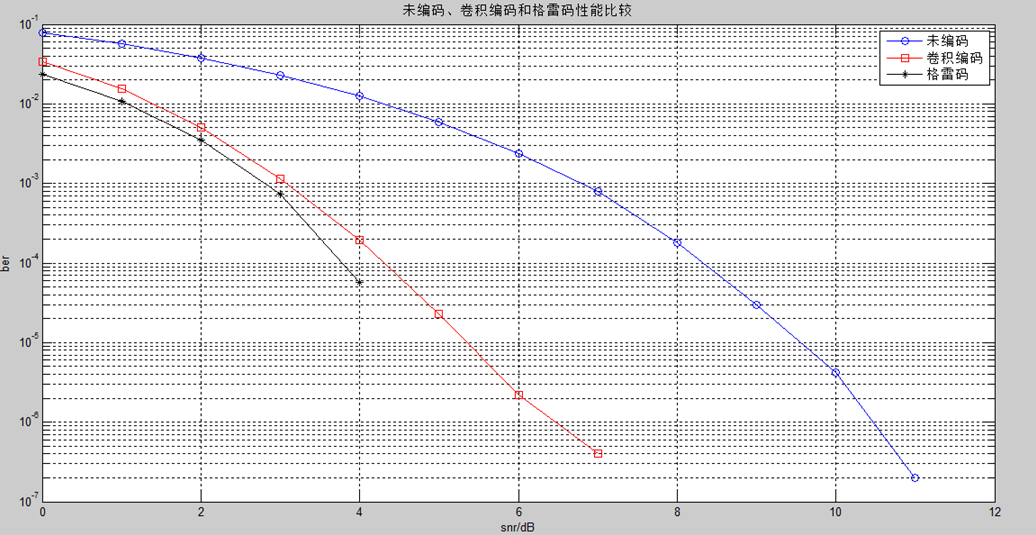``````以上仿真结果可知：
1、未编码、卷积编码和格雷码三种编码，经PSK调制后加AWGN（高斯白噪声），在经过解调和解码得出来的码字误码率都随着信噪比的增加而减小。
2、有仿真图的三条曲线可知，三种编码在PSK调制，外加AWGN后的性能好坏依次是：格雷码 > 卷积编码 > 未编码。
``````

1、卷积码的编译码原理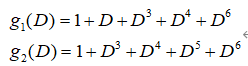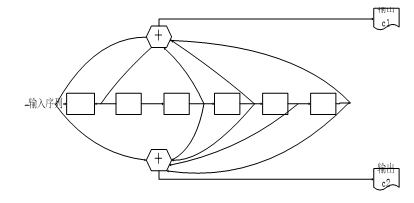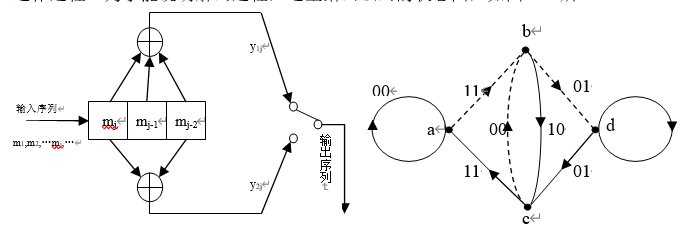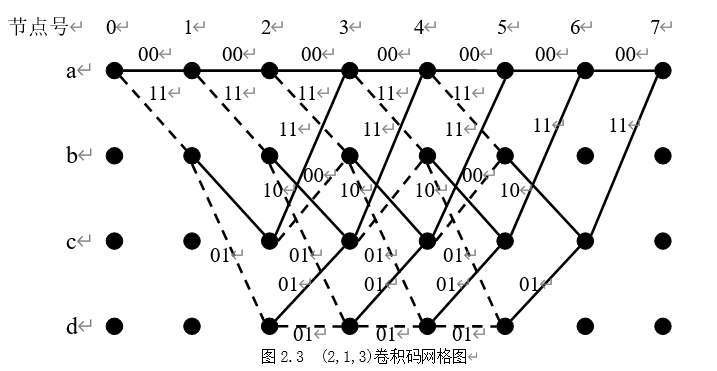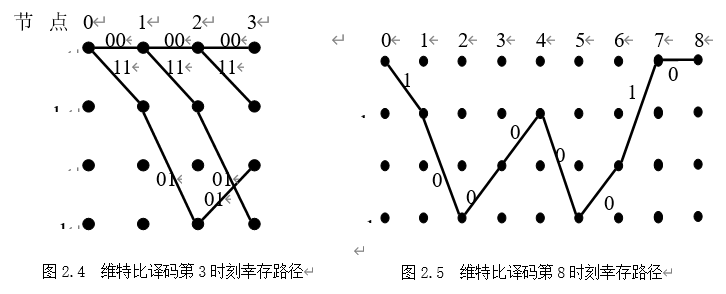2、格雷码的编译码原理

（2）根据伴随式s(x)找出对应的估值错误图样 ；
（3）计算 ，得到估计码字 。若 ,则译码正确，否则，若 ，则译码错误。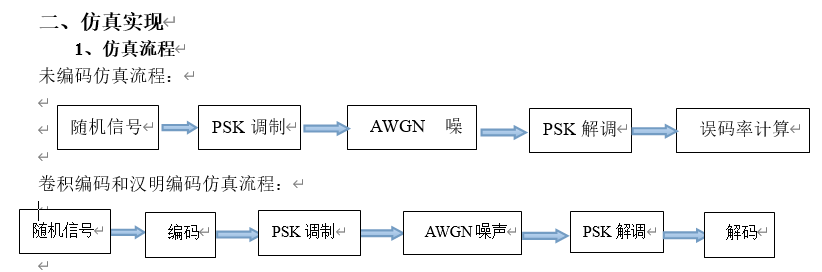``````function bianmaa
cycl=50;
snr=0:1:12;
msg=randint(1,100000);
ber0=zeros(1,length(snr));
ber1=zeros(1,length(snr));
trellis=poly2trellis(3,[5 7]);
for n=1:cycl
for k=1:length(snr)
modbit0=pskmod(msg,2);
y0=awgn(modbit0,snr(k),'measured');
demmsg0=pskdemod(y0,2);
recode0=reshape(demmsg0',1,[]);
[num0,rat0]=biterr(recode0,msg);
ber0(n,k)=rat0;
end
end
ber0=mean(ber0);
code=convenc(msg,trellis);
modbit1=pskmod(code,2);
for n=1:cycl
for k=1:length(snr)
y1=awgn(modbit1,snr(k),'measured');
demmsg1=pskdemod(y1,2);
recode1=reshape(demmsg1',1,[]);
tblen=5;
decoded1=vitdec(recode1,trellis,tblen,'cont','hard');
[num1,rat1]=biterr(double(decoded1(tblen+1:end)),msg(1:end-tblen));
ber1(n,k)=rat1;
ber1(k)=rat1;
end
end
ber1=mean(ber1);
msg1=randint(70000,1,2);
code=encode1(msg,24,12,);
modbit2=pskmod(code,2);
for k=1:length(snr)
y2=awgn(modbit2,snr(k),'measured');
demmsg2=pskdemod(y2,2);
recode=reshape(demmsg2',1,[]);
decodebit=decode1(recode,24,12);
error2=(decodebit~=msg1');
errorbits=sum(error2);
ber2(k)=errorbits/length(msg1);
end
semilogy(snr,ber0,'b-o',snr,ber1,'r-s',snr,0.8*ber2,'k-*');
xlabel('snr/dB');
ylabel('ber');
legend('未编码','卷积编码','格雷码');
title('未编码、卷积编码和格雷码性能比较');
grid on
``````